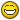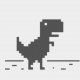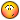# LISP for creating text label (numbering) of the sheet of cadastral map

## Recommended PostsHello all,

I am new to AutoLISP, and I have an assignment to make a lisp which would calculate and write number of the sheet of cadastral map (which is defined by scale and coordinates). I'd like the sheet scale in which the number is calculated to be 1:1000 (more advanced option - to be able to choose). Numbering is defined on this link : http://listovi.dgu.hr/vezaizmedjupodjela.html

(I'm from Croatia)

Any help would be great, thanks.##### Share on other sitesSimple maths if you pick a point you can work out the grid values as you know the starting base point of the grids. eg x=435,000 divide by start grid is 200,000 grid spacing is 25,000 =integer (435,000-200,000)/25,000) = grid number 9

##### Share on other sitesjust a starting point..

```(defun c:test (/ l \$) ; scale ,x-grid, y-grid

(setq	l '((250000 150000 100000)
(100000 60000 40000)
(50000 30000 20000)
(25000 15000 10000)
(10000 6000 4000)
(5000 3000 2000)
(2000 1200 800)
(1000 600 400)
(500 300 200)
)
) ;_ end of setq
(if (progn (initget "250k 100k 50k 25k 10k 5k 2k 1k 0.5k")
(setq \$ (getkword "\nSelect Scale 1:<250k/100k/50k/25k/10k/5k/2k/1k/0.5k>? : "))
) ;_ end of progn

[color="darkgreen"];what kind of labelling? elaborate more?[/color]

[color="darkgreen"] ;Here's the example only shows the grid factor if correct[/color]
(apply 'strcat
(mapcar ' ' ((a b)(strcat a (itoa b))) '("Scale= " " | X-Grid= " " | Y-Grid= ")
(assoc (* (atoi (vl-string-left-trim "k" \$)) 1000) l)))
)
[color="darkgreen"]

;if you wanna label the grid coordinates?
;the easiest way without lisp coding is creating Mtext with FIELD xy-coordinates, then array with it's xy-grid factor[/color]

(princ "\oops!")
) ;_ end of if
(princ)
) ;_ end of defun
```

##### Share on other sitesThanks for the responses, the hierarchy behind labeling is in the .dwg's attached. As I'm completely new to this, I'm just catching up with the basics of lisp programming, my professor told me to use math on this, but I'm still struggling on how to combine the mathematic solution with programming. I don't need to label the grid coordinates, I just want to get a text which contains the label of the grid area (rectangle) depending on the picked point coordinates and scale, eg. "1-2-55-105-9", in which 105-9 stands for 105th (101st being the 1st; 100 is added not to mix rows and columns) row and 9th column in the scale of 1:50000; 55 stands for 55th area (or sheet - i'm not sure which terminology to use) in the scale of 1:2000 (50k rectangle is divided into 625 2k rectangles); and 1-2 stands for the area in the scale of 1:1000 (number 1 presenting that this is a numbering in the scale of 1:1000 (1k), and number 2 presenting a second area in the scale of 1:1000; 2k rectangle is divide into 4 1k rectangles). This is better explained in .dwg's. Sorry for the long read.50k (25k-5k, 10k, 2k-1k-0.5k).dwg

100k.dwg

250k.dwg

##### Share on other sites...I just want to get a text which contains the label of the grid area (rectangle) depending on the picked point coordinates and scale..

hi, try this function

```[color="darkgreen"];|
function - [b]MAP-SHEET[/b]
argument - Type
------------------------
n	- scale, number
mX      - max X, number
mY	- max Y, number
\$	- suffix, string
-------------------------
Return value:
A string
---------------------------
example	:
(MAP-SHEET 10000 30000 20000 "105-9")
n, 10000 = scale 1:10000
mX, 30000 = maximum X range of sheet
mY, 20000 = maximum Y range of sheet
\$, "105-9" = suffix of upper level sheet
returns: suffix of newly selected sheet|;[/color]

(defun [color="blue"][b]MAP-SHEET[/b][/color] (n mX mY \$ / ls % d l p p1 p2 k)
[color="green"];hanhphuc 12.12.2016[/color]

(if (setq ls '((250000 150000 100000)
(100000 60000 40000)
(50000 30000 20000)
(25000 15000 10000)
(10000 6000 4000)
(5000 3000 2000)
(2000 1200 800)
(1000 600 400)
(500 300 200)
)
l  (assoc n ls)
p1 (getpoint (strcat "\nPick upper left corner of sheet - [M 1:" (itoa n) "] "))
)
(progn (princ "\nHover the mouse over & pick a box.. \n")
(while (and p1 (setq p (grread t 1 0)) (= 5 (car p)) (setq p2 (cadr p)))
(princ (strcat "\rSHEET " (cond (%)(""))"          "))
(setq d  (mapcar '- p2 p1)
ls (reverse (mapcar '+ '(1 -101) (mapcar ''((x y) (fix (/ x y))) d (cdr l))))
k  (mapcar '+ '(1 -1) (mapcar ''((x y) (fix (/ x y))) d (cdr l)))
k  (- (* (1+ (cadr k)) (/ mY (caddr l))) (car k))
%  (if
;(vl-some ''((x) (or (minusp x) (> (abs x) 600000))) (list (car d) (- (cadr d)))); for Square only
(or (> (abs (car d)) mX) (> (abs (cadr d)) mY) (minusp (car d)) (minusp (- (cadr d))))
"\rOut of range!!           "
(cond (\$ (apply 'strcat (append (mapcar 'itoa (list (/ (car l) 1000) k)) (list "-" \$))))
((vl-string-right-trim
"-"
(apply 'strcat
(mapcar ''((x) (strcat (itoa x) "-")) (cons (/ (car l) 1000) (mapcar 'abs ls)))
)
)
)
)
)
)
)
)
)
(if (and % (/= % "\rOut of range!!           "))
(substr % (+ 2 (vl-string-search "-" %)))
""
)
)
```

Example call

```[b]
[color="red"]([/color][color="blue"]MAP-SHEET[/color] [color="darkgreen"]10000 30000 20000[/color] [color="magenta"]"105-9"[/color][color="red"])[/color][/b]

Pick upper left corner of sheet - [M 1:10000]
Hover the mouse over & pick a box..
SHEET 10-7-105-9  [color="red"];<-- Dynamically displaying in command line upon moving the mouse[/color]

```

or make defun

```
(defun c:MAP50K	nil
(if (= (getvar 'dwgname) "50k (25k-5k, 10k, 2k-1k-0.5k).dwg")
([color="blue"]MAP-SHEET[/color] 50000 600000 600000 [color="red"]nil[/color])
)
(princ)
)

(defun c:MAP5K nil
(if (= (getvar 'dwgname) "50k (25k-5k, 10k, 2k-1k-0.5k).dwg")
([color="blue"]MAP-SHEET[/color]
[color="red"]5000[/color] [color="green"];final step 1:5000[/color]
15000
10000
([color="blue"]MAP-SHEET[/color] [color="green"];2nd step 1:25000[/color]
[color="red"]25000[/color]
30000
20000
([color="blue"]MAP-SHEET[/color]
[color="red"]50000[/color]
600000
600000 [color="red"]nil[/color])
) [color="green"]; 1st step 1:50000[/color]
)
)
(princ)
)
```

HTH

Edited by hanhphuc
color & comment

##### Share on other sitesThank you...sir hanh phuc

This is not my problem ... but in view of the requirements of the first I was not obvious (english not enough to present my ideas) but also I am a surveyor should be able to understand all lisp for creating text label (numbering) of the sheet of cadastral map need to map 1/5000, 1/2000, 1/1000, and 1/500-specific

if so wrong ... please forgive

##### Share on other sitesThank you...sir hanh phuc This is not my problem ... but in view of the requirements of the first I was not obvious (english not enough to present my ideas) but also I am a surveyor should be able to understand all lisp for creating text label (numbering) of the sheet of cadastral map need to map 1/5000, 1/2000, 1/1000, and 1/500-specific

if so wrong ... please forgive

i'm not sure does this thread help you?In fact i'm not familiar about the mapping labeling (it looks weird to me).

my understanding as OP quoted:

...I don't need to label the grid coordinates, I just want to get a text which contains the label of the grid area (rectangle) depending on the picked point coordinates and scale, eg. "1-2-55-105-9"....

i'm also learning something new from OP's info. my concept is based on what his requirement just with dynamic output. In fact you can try entmake'ing TEXT with minor tweak

For automated labeling, suggestion: defun your new function eg: vuong-sheet, remove grread thing in code, add extra arguments pt bp

```;example:
(vuong-sheet [color="red"]pt bp[/color] n mX mY \$ ) ;where pt= supplied any point, bp= is top left known coordinates of selected map,
then you can iterate in a loop etc..```

good luck

##### Share on other sitessorry sir hanh phuc!

I'm busy too few today...

##### Share on other sitesDear hahnphuc, your program is great, but I think BIGAL's post explains the method which should be used in programming. The base points of the grid are X = 200000.00 Y = 5170000.00 - north-west, and X = 800000.00 Y = 4570000.00 - south-east. I'd like the program to do the following: when I pick a coordinate, eg (X = 409354.53 Y = 4937853.78), the program calculates the values of the grid (meaning the row and column), eg. for X coordinate - (409354-200000)/150000=2 -> the column is number 2, for Y coordinate (5170000-4937853)/10000=3 -> the row is number 3 (from north to south); so the label which the program should write would be "250-103-2". I hope this explains it, rgds

##### Share on other sitesA grid would normally start at whole numbers rather than a random point, here is an example of repeated text at a spacing.

```(setq x 0.0)
(setq y 0.0)
(setq inc (Getreal "Enter spacing say 1000"))
(repeat (getint "Enter how many grids")
(command "text" (list x y) "" "" (rtos x 2 0))
(setq x (+ x inc))
)
```

##### Share on other sitesHi BIGAL, could you maybe help me with this routine, it's for labeling in M:1:250K? (I'm new to lisp programming, so it's full of errors, probably)

```(defun c:gisprog ()
(setq x 200000.00)
(setq y 5170000.00)
(setq pt ( getpoint "\nPick a point : "))
(multiple-value-bind (q r) (floor (- (car pt) 200000) 150000) q)
(setq column (+ q 1))
(multiple-value-bind (q r) (floor (- 5170000 (cadr pt)) 100000) q)
(setq row (+ q 1)
princ (strcat "\n250-" rtos (+ row 100) "-" rtos column)
)
)```

##### Share on other sitesYou have used true LISP programming functions not Autocad Lisp which is a subset of the LISP programming language. Floor and multiple-value-bind do not exist.

Go back to what I posted as a start you need to look at functions like FIX to round the numbers.

##### Share on other sites...I'd like the program to do the following: when I pick a coordinate, eg (X = 409354.53 Y = 4937853.78), the program calculates the values of the grid (meaning the row and column)..

actually the previous function does the same concept, but results just echo in the command line ,without label

```(MAP-SHEET 250000 600000 600000 nil)
```

however, as mentioned in post#7 slightly modify the function which user can supply more argument to be more generic

code updated v:1.0

hanhphuc 18.01.2017

```[color="darkgreen"];|argument - Type
---------------------------
pt	- specified point
bp	- Base coordinates
n	- scale, number
mX      - max X, number
mY	- max Y, number
\$	- suffix, string
-------------------------
Return value:
A string
---------------------------
example	:
(MAP-SHEET[color="red"]:[/color] '(409354.53 4937853.7) '(200000 5170000)  250000 60000 60000 nil)
pt,'(409354.53 4937853.7) = specified point inside the required sheet
bp, '(200000 5170000) = base coordinates of sheet at upper left corner, list
n, 250000 = scale 1:10000
mX, 600000 = maximum X range of sheet
mY, 600000 = maximum Y range of sheet
\$, nil = suffix of upper level sheet
returns: list, (suffix x y z )
example call:
(MAP-SHEET: pt bp 250000 600000 600000 nil)
'("102-2" 418370.0 5.03597e+006 0.0)
|;
[/color]
(defun [color="blue"][b]MAP-SHEET:[/b][/color] (pt bp n mX mY \$ / ls d l p k) ;hanhphuc 20.12.2016
(if (setq ls '((250000 150000 100000)
(100000 60000 40000)
(50000 30000 20000)
(25000 15000 10000)
(10000 6000 4000)
(5000 3000 2000)
(2000 1200 800)
(1000 600 400)
(500 300 200)
)
l  (assoc n ls)
)
(progn (setq d  (mapcar '- pt bp)
ls (reverse (mapcar '+ '(1 -101) (mapcar ''((x y) (fix (/ x y))) d (cdr l))))
k  (mapcar '+ '(1 -1) (mapcar ''((x y) (fix (/ x y))) d (cdr l)))
k  (- (* (1+ (cadr k)) (/ mY (caddr l))) (car k))
%  (if	(or (> (abs (car d)) mX) (> (abs (cadr d)) mY) (minusp (car d)) (minusp (- (cadr d))))
"\rOut of range!!           "
(cond (\$ (apply 'strcat (append (mapcar 'itoa (list (/ (car l) 1000) k)) (list "-" \$))))
((vl-string-right-trim
"-"
(apply 'strcat
(mapcar ''((x) (strcat (itoa x) "-")) (cons (/ (car l) 1000) (mapcar 'abs ls)))
)
)
)
)
)
)
(cons
(if (and % (/= % "\rOut of range!!           "))
(substr % (+ 2 (vl-string-search "-" %)))
""
)
pt
)
) ; progn
)
)
```

example applied in labeling function , map-label

```[color="darkgreen"]
;|
example call:
(map-label
"250K" ; str - message for sheet selection
'(200000.00  5170000.00 ) ; p1 - coordinates of sheet at upper left corner
1 ; f - repeating flag, 1 or 0
7000 ; text height
250000 ; scale factor 1:250000
600000 ; maximum X range of sheet
600000 ;maximum Y range of sheet
nil ; suffix of upper level sheet or N/A
)
|;
[/color]
(defun [color="blue"]map-label[/color] (str p1 f h n mX mY \$ / l p2)
(prompt (strcat "\nSpecify point " str "\n"))
(eval
(cons (if	(zerop f)
'progn
'while
)
'((while
(and (setq p (grread t 1 0)) (= 5 (car p)) (setq p2 (cadr p)))
(setq l ([color="blue"]MAP-SHEET:[/color] p2 p1 n mX mY \$))
(if
(/= (car l) "")
(princ (strcat "\rSHEET " (setq str (itoa (/ n 1000))) "-" (car l) "       "))
(prompt "\rOut of range!          ")
)
)
(entmakex
(mapcar ''((a b) (cons a b)) '(0 1 10 40 50)
(list "TEXT" (strcat str "-" (car l)) (trans (cdr l) 1 0)
h (angle '(0. 0. 0.) (getvar 'ucsxdir))
)
)
)
)
)
)
(car l)
)
```

look at the example for map250K ,map50K, map10K, you can simply modify the argument for other sheets

```[color="green"];with '(200000.00  5170000.00 ) known base coordinates without user picking[/color]
(defun c:map250K nil
(if (= (getvar 'dwgname) "250k.dwg")
([color="blue"]map-label[/color] "Sheet [M 1:250000]"
[color="red"] '(200000.00  5170000.00 )[/color] [color="green"];known upper left corner[/color]
1
7000
[color="red"]250000[/color]
600000
600000
nil)
)
(princ)
)

[color="green"];if corner unknown, user pick example[/color]
(defun c:map50K	(/ pt)
(if (= (getvar 'dwgname) "50k (25k-5k, 10k, 2k-1k-0.5k).dwg")
(and (setq pt (getpoint "\nPick Upper Left corner of sheet - [M 1: 50000 ]"))
([color="blue"]map-label[/color] "Sheet [M 1:50000]"
pt 1 2000 [color="red"]50000[/color] 600000 600000 nil)
)
)
(princ)
)

[color="green"];if known base point of 2 different sheets[/color]
(defun c:map10K	nil
(Alert "\nSelect sheet in [M 1:50000] \nthen specify label insertion point in [M 1:10000].. ")
(if (= (getvar 'dwgname) "50k (25k-5k, 10k, 2k-1k-0.5k).dwg")
([color="blue"]map-label[/color] "Sheet [M 1:10000]"
[color="red"]'(928187.08 5276613.90)[/color] [color="green"];for sheet 1:10K[/color]
1
500
[color="red"]10000[/color]
30000
20000
([color="blue"]map-label[/color] "Sheet [M 1:50000]"
[color="red"]'(200000.00  5170000.00 )[/color] [color="green"];for sheet 1:50k [/color]
0
2000
[color="red"]50000[/color]
600000
600000
nil))

)
(princ)
)
```

quite busy since last december, good luck

##### Share on other sitesThank you all for your help, I've managed to write a lisp which works quite well for my requirements, so I'm putting it here for you to see..

vuongsurvey, if you need something like this, I'd be glad to help you out and modify it for your needs..```(defun c:1K ()
(setq x 200000.00)
(setq y 5170000.00)
(setq pt ( getpoint "\nPikni točku : "))
(setq column (+ (fix (/ (- (car pt) x) 30000 ) ) 1 ) )
(setq row (+ (fix (/ (- y (cadr pt) ) 20000 ) ) 1 ) )
(setq x2 (+ x (* (fix (- column 1)) 30000) ))
(setq y2 (- y (* (fix (- row 1)) 20000) ))
(setq column2 (fix (/ (- (car pt) x2) 1200 ) ))
(setq row2 (+ (fix (/ (- y2 (cadr pt))  800 ) ) 1 ) )
(setq x3 (+ x2 (* (- column2 1) 1200)))
(setq y3 (- y2 (* (- row2 1) 800)))
(setq column3 (fix (/ (- (car pt) x3) 600 ) ))
(setq row3 (+(fix (/ (- y3 (cadr pt))  400 ) ) 1 ) )
(setq x4 (+ x3 (* (- column3 1) 600)))
(setq y4 (- y3 (* (- row3 1) 400)))
(setq nom (strcat "1-" (itoa (- (+ column3 (* (- row3 1) 2)) 1)) "-" (itoa (+ (+ column2 (* (- row2 1) 25)) 1)) "-" (itoa (+ row 100)) "-" (itoa column)))
(setq ptrec1 (list (+ x4 1200) (- y4 400) 0))
(setq ptrec2 (list (+ x4 600) y4 0))
(setq oldosmode (getvar "osmode"))
(setvar "osmode" 0)
(command "_rectangle" "_from" ptrec1 "@0,0" "_from" ptrec2 "@0,0")
(setvar "osmode" oldosmode)
princ (strcat "1-" (itoa (- (+ column3 (* (- row3 1) 2)) 1)) "-" (itoa (+ (+ column2 (* (- row2 1) 25)) 1)) "-" (itoa (+ row 100)) "-" (itoa column))
(if (not (tblsearch "Layer" "Nomenklatura_1K"))
(command "-layer" "m" "Nomenklatura_1K" "")
)
(entmake
(list
'(0 . "MTEXT")
'(100 . "AcDbEntity")
'(100 . "AcDbMText")
(cons 10 ptrec2)
(cons 71 1) ; 1 = Top Left
(cons 50 0.0) ; rotation angle
(cons 040 20)
(cons 8 "Nomenklatura_1K")
(cons 1 nom)
)
)
)```

## Join the conversation

You can post now and register later. If you have an account, sign in now to post with your account.
Note: Your post will require moderator approval before it will be visible.×   Pasted as rich text.   Paste as plain text instead

Only 75 emoji are allowed.